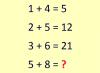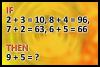BRAIN TEASERSBrain Teasers User Profile

# Paulynom 101

rank
613
points
2
See full ranking list
short ranking list
 611 Sub Roy 2 612 TD Games 2 613 Paulynom 101 2 614 Kirti Jai Gupta 2 615 James Monica 2
 Calculate 5+8 If 1+4=5, 2+5=12 and 3+6=21 then 5+8=?What is 9 + 5? If 2+3=10, 8+4=96, 7+2=63, 6+5=66 then 9+5=?